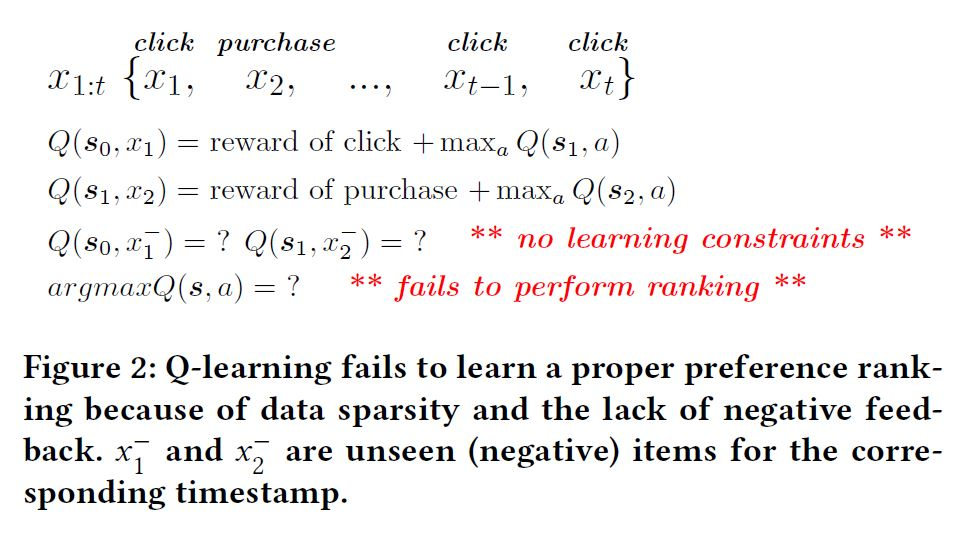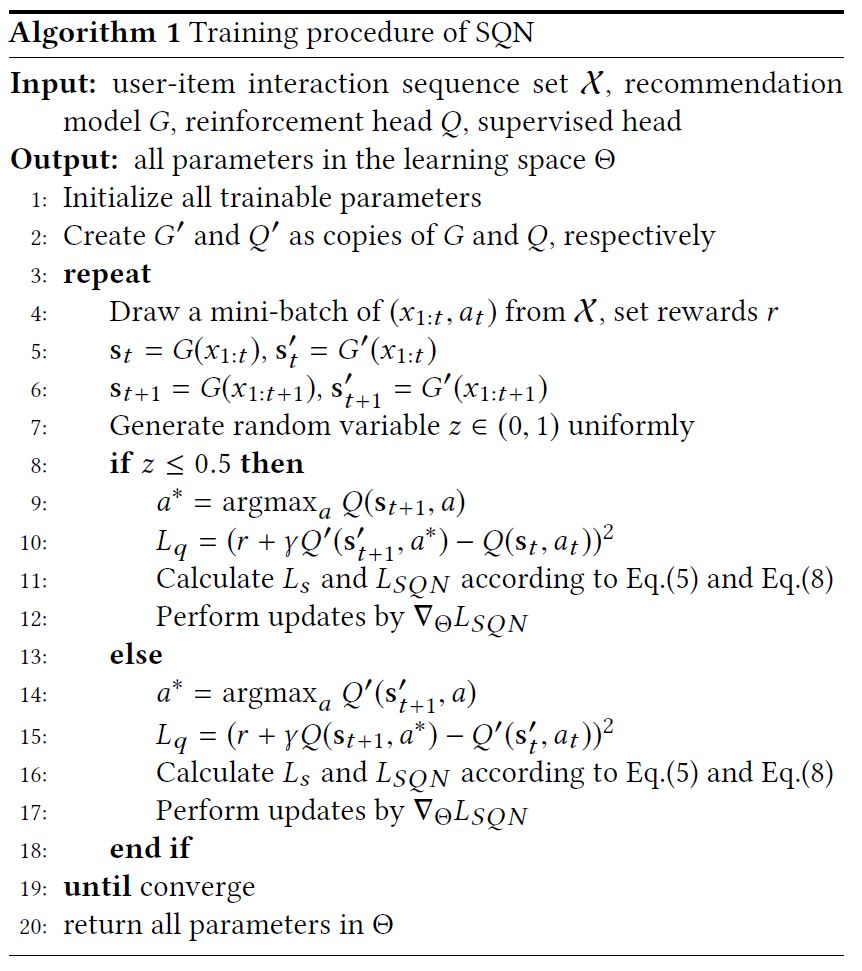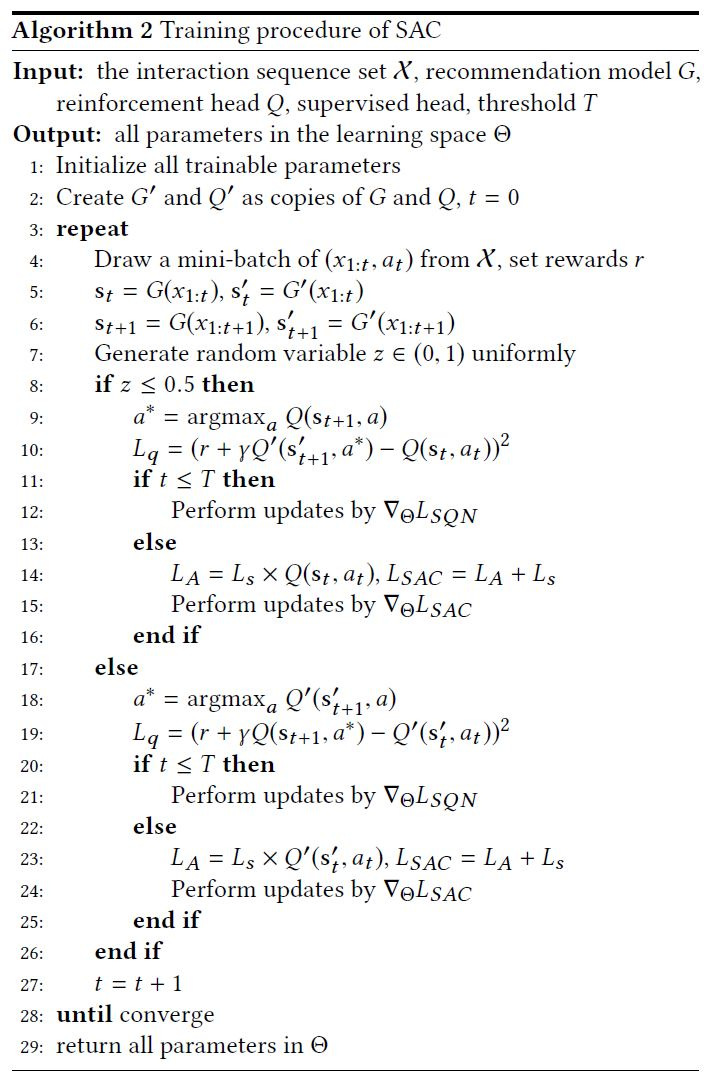## 论文信息

« Self-Supervised Reinforcement Learning for Recommender Systems »

SIGIR-2020

session-based or sequential recommendation

## 架构图## 过程

### 强化学习建模

S

$s_{t} = G(x_{1:t})$

$x_{1:t}$ 是用户行为序列, G 是编码器(例如 GRU 等)

A

R

### 为什么不能直接用强化学习做推荐### SQN

#### 训练阶段

input: $(x_{1:t} , a_t )$

$L_{SQN} = L_{s} + L_{q}$

##### 第一部分

$L_{s}=-\sum_{i=1}^{n} Y_{i} \log \left(p_{i}\right)$

$p_{i}=\frac{e^{y_{i}}}{\sum_{i^{\prime}=1}^{n} e^{y_{i} \prime}}$

$p_i$ 为物品的 classification distribution

##### 第二部分

$L_{q}=\left(r\left(\mathrm{~s}{t}, a{t}\right)+\gamma \max {a^{\prime}} Q\left(\mathrm{~s}{t+1}, a^{\prime}\right)-Q\left(\mathrm{~s}{t}, a{t}\right)\right)^{2}$

$Q\left(s_{t}, a_{t}\right)=\delta\left(s_{t} \mathbf{h}{t}^{T}+b\right)=\delta\left(G\left(x{1: t}\right) \mathbf{h}_{t}^{T}+b\right)$### SAC

$L_{A}=L_{s} \cdot Q\left(\mathrm{~s}{t}, a{t}\right)$## 实验

1. 图4中可以看到调整购买与点击奖励比例对购买或点击 NDCG 的直接影响

2. 图7, 8中可以看出直接用强化学习进行推荐性能很差